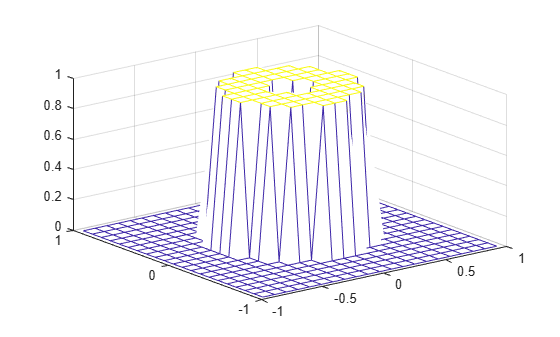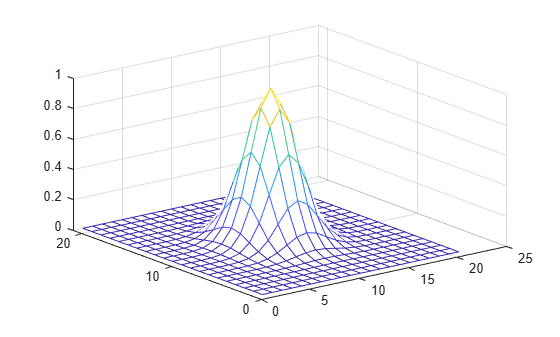fwind2

2-D FIR filter using 2-D window method

Description

The fwind2 function designs 2-D FIR filters using the window method. fwind2 uses a 2-D window specification to design a 2-D FIR filter based on the desired frequency response. fwind2 works with 2-D windows only. Use fwind1 to create a 2-D FIR filter from a 1-D window.

You can apply the 2-D FIR filter to images by using the filter2 function.

example

h = fwind2(Hd,win) creates a 2-D FIR filter h by using an inverse Fourier transform of the desired frequency response Hd and multiplication by the window win.

h = fwind2(f1,f2,Hd,win) lets you specify the desired frequency response Hd at arbitrary frequencies f1 and f2 along the x- and y-axes.

Examples

collapse all

This example shows how to design an approximately circularly symmetric two-dimensional bandpass filter using a 2-D window method.

Create the frequency range vectors f1 and f2 using freqspace. These vectors have length 21.

[f1,f2] = freqspace(21,'meshgrid');

Compute the distance of each position from the center frequency.

r = sqrt(f1.^2 + f2.^2);

Create a matrix Hd that contains the desired bandpass response. In this example, the desired passband is between 0.1 and 0.5 (normalized frequency, where 1.0 corresponds to half the sampling frequency, or $\pi$ radians).

Hd = ones(21);
Hd((r<0.1)|(r>0.5)) = 0;

Display the ideal bandpass response.

colormap(parula(64))
mesh(f1,f2,Hd)Create a 2-D Gaussian window using fspecial. Normalize the window.

win = fspecial('gaussian',21,2);
win = win ./ max(win(:));

Display the window.

mesh(win)Using the 2-D window, design the filter that best produces the desired frequency response

h = fwind2(Hd,win);

Display the actual frequency response of this filter.

freqz2(h)Input Arguments

collapse all

Desired frequency response at equally spaced points in the Cartesian plane, specified as a numeric matrix. For accurate results, create Hd by using the freqspace function.

Data Types: single | double | int8 | int16 | int32 | int64 | uint8 | uint16 | uint32 | uint64

2-D window, specified as a numeric matrix.

Data Types: single | double

Desired frequency along the x-axis. The frequency vector should be in the range [-1, 1], where 1.0 corresponds to half the sampling frequency, or π radians.

Data Types: single | double

Desired frequency along the y-axis. The frequency vector should be in the range [-1, 1], where 1.0 corresponds to half the sampling frequency, or π radians.

Data Types: single | double

Output Arguments

collapse all

2-D FIR filter, returned as a numeric matrix of the same size as win.

Data Types: double

Algorithms

fwind2 computes h using an inverse Fourier transform and multiplication by the two-dimensional window win.

${h}_{d}\left({n}_{1},{n}_{2}\right)=\frac{1}{{\left(2\pi \right)}^{2}}{\int }_{-\pi }^{\pi }{\int }_{-\pi }^{\pi }{H}_{d}\left({\omega }_{1},{\omega }_{2}\right){e}^{j{\omega }_{1}{n}_{1}}{e}^{j{\omega }_{2}{n}_{2}}d{\omega }_{1}d{\omega }_{2}$

$h\left({n}_{1},{n}_{2}\right)={h}_{d}\left({n}_{1},{n}_{2}\right)w\left({n}_{1},{n}_{2}\right)$

 Lim, Jae S., Two-Dimensional Signal and Image Processing, Englewood Cliffs, NJ, Prentice Hall, 1990, pp. 202-213.